Test: Building With Bricks - 5

# Test: Building With Bricks - 5 - Class 4

Test Description

## 20 Questions MCQ Test Mathematics for Class 4: NCERT - Test: Building With Bricks - 5

Test: Building With Bricks - 5 for Class 4 2023 is part of Mathematics for Class 4: NCERT preparation. The Test: Building With Bricks - 5 questions and answers have been prepared according to the Class 4 exam syllabus.The Test: Building With Bricks - 5 MCQs are made for Class 4 2023 Exam. Find important definitions, questions, notes, meanings, examples, exercises, MCQs and online tests for Test: Building With Bricks - 5 below.
Solutions of Test: Building With Bricks - 5 questions in English are available as part of our Mathematics for Class 4: NCERT for Class 4 & Test: Building With Bricks - 5 solutions in Hindi for Mathematics for Class 4: NCERT course. Download more important topics, notes, lectures and mock test series for Class 4 Exam by signing up for free. Attempt Test: Building With Bricks - 5 | 20 questions in 40 minutes | Mock test for Class 4 preparation | Free important questions MCQ to study Mathematics for Class 4: NCERT for Class 4 Exam | Download free PDF with solutions
 1 Crore+ students have signed up on EduRev. Have you?
Test: Building With Bricks - 5 - Question 1

### Karan plans to cover the boundary of a park with tiles what should Karan know to make sure he buys enough tiles?

Detailed Solution for Test: Building With Bricks - 5 - Question 1

Karan should know about the perimeter of the park to make sure he buys enough tiles.

Test: Building With Bricks - 5 - Question 2

### Varun made a shape by folding the given along dotted lines. Which shape did Varun make?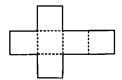Detailed Solution for Test: Building With Bricks - 5 - Question 2

After folding along dotted lines, a cube is formed.

Test: Building With Bricks - 5 - Question 3

### Sahil has a field in the shape of rectangle. The length of the field is twice its breadth. The breadth is 80 m. Find the perimeter of the field

Detailed Solution for Test: Building With Bricks - 5 - Question 3

Breadth of the field = 80 m Length of the field = 2 × 80 = 160m Perimeter of the field = 2 (length + breadth) =2(160 + 80)=2 × 240 = 480m

Test: Building With Bricks - 5 - Question 4

An ant is crawling from point A to point B using the staircase. How much distance will it crawl to reach point B?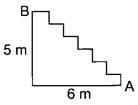Detailed Solution for Test: Building With Bricks - 5 - Question 4

We have,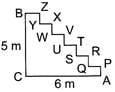AP + QR + ST + UV + WX + YZ = BC = 5 m PQ + RS + TU + VW + XY + ZB = AC = 6 m So, distance crawl by the ant = (5 + 6) m = 11 m

Test: Building With Bricks - 5 - Question 5

For a project Simmi cut a piece of paper into a shape with 4 sides, but none of them are of the same length. What is the shape of the paper?

Detailed Solution for Test: Building With Bricks - 5 - Question 5

Since there are 4 sides but none of them are of same length, therefore, the shape of the paper is quadrilateral.

Test: Building With Bricks - 5 - Question 6

The given figure (not drawn to scale) is made up of 6 identical squares. The perimeter of the figure is 126 cm. What is the length of each side of the square?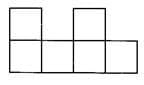Detailed Solution for Test: Building With Bricks - 5 - Question 6

Let side of a square be X cm. Perimeter of the square = 126 cm ⇒ 14X = 126 ⇒ X = 9 Hence, side of the square is 9 cm.

Test: Building With Bricks - 5 - Question 7

How many of the following figures are symmetrical?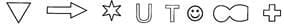Detailed Solution for Test: Building With Bricks - 5 - Question 7

All the figures are symmetrical.

Test: Building With Bricks - 5 - Question 8

The given figure is obtained by placing a rectangle on top of another identical rectangle. The length of a rectangle is 5 cm and its breadth is 2 cm. Find the perimeter of the figure.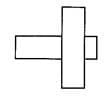Detailed Solution for Test: Building With Bricks - 5 - Question 8

Perimeter of the figure = AB + BC + CD + DE + EF + FG + GH + HI + IJ + JK + KL + LA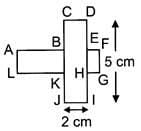= (AB + CD + FE) + (BC + AL + JK) + (DE + FG + HI) + (GH +IJ + KL)

Test: Building With Bricks - 5 - Question 9

Each of the figures below is made up of identical 2 cm squares. Which one of the figures has the greatest perimeter?

Detailed Solution for Test: Building With Bricks - 5 - Question 9

Figure C has the greatest perimeter.

Test: Building With Bricks - 5 - Question 10

The given figure (not drawn to scale) is made up of a rectangle, a square and a triangle. Find the perimeter of the figure.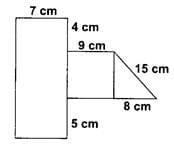Detailed Solution for Test: Building With Bricks - 5 - Question 10

We have,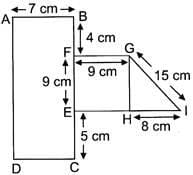AD = BF + FE + EC
=4 + 9 + 5 =18 cm
Also, AB = DC = 7 cm FG = EH = 9 cm Now, perimeter of the given figure = AB + BF + FG + GI + IH + HE + EC + CD + DA  = 7 + 4 + 9 + 15 + 8 + 9 + 5 + 7 + 18 = 82 cm

Test: Building With Bricks - 5 - Question 11

Which of the following is the name of the distance between the centre of the circle to any point on it?

Detailed Solution for Test: Building With Bricks - 5 - Question 11

Radius is the distance between the centre of the circle to any point on it.

Test: Building With Bricks - 5 - Question 12

Find the word that fills the blank.
A_____ is a chord that Passes through the centre of the circle.

Detailed Solution for Test: Building With Bricks - 5 - Question 12

Diameter is a chord that passes through the centre of the circle.

Test: Building With Bricks - 5 - Question 13

What is the total length of the boundary of a circle?

Detailed Solution for Test: Building With Bricks - 5 - Question 13

Total length of the boundary of a circle is its circumference.

Test: Building With Bricks - 5 - Question 14

What is an arc representing half of a circle?

Detailed Solution for Test: Building With Bricks - 5 - Question 14

A semi-circle represents half of a circle.

Test: Building With Bricks - 5 - Question 15

What do you call the line that divides an object or a figure into two identical halves?

Detailed Solution for Test: Building With Bricks - 5 - Question 15

A line of symmetry divides an object or a figure into two identical halves.

Test: Building With Bricks - 5 - Question 16

How many end points does a line segment have?

Detailed Solution for Test: Building With Bricks - 5 - Question 16

A line segment has 2 end points.

Test: Building With Bricks - 5 - Question 17

How many lines can be drawn through two given points?

Detailed Solution for Test: Building With Bricks - 5 - Question 17

Through two given points, we can draw only 1 line.

Test: Building With Bricks - 5 - Question 18

What is the other name of a rectangle with four equal sides?

Detailed Solution for Test: Building With Bricks - 5 - Question 18

A square is a rectangle with 4 equal sides.

Test: Building With Bricks - 5 - Question 19

What is the number of triangles in the given figure?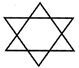Detailed Solution for Test: Building With Bricks - 5 - Question 196 small triangles + 2 big triangles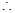Number of triangles = 8

Test: Building With Bricks - 5 - Question 20

Which of the following points is not in line with the others?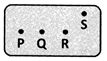Detailed Solution for Test: Building With Bricks - 5 - Question 20

S is not in line with P, Q and R.

## Mathematics for Class 4: NCERT

25 videos|84 docs|41 tests
Information about Test: Building With Bricks - 5 Page
In this test you can find the Exam questions for Test: Building With Bricks - 5 solved & explained in the simplest way possible. Besides giving Questions and answers for Test: Building With Bricks - 5, EduRev gives you an ample number of Online tests for practice

## Mathematics for Class 4: NCERT

25 videos|84 docs|41 tests